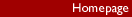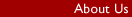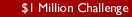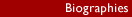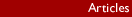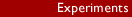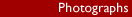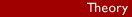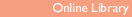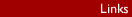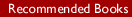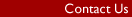ONLINE LIBRARY Book: "Intelligence Behind the Universe!" Author: Ronald D. Pearson B.Sc (Hons) Availability: From Michael Roll Contents / Previous Chapter / Next Chapter - Chapter 5 - Digression Negative Energy States & Gravitation ___________________________________________ 5.1 Problems Raised by Gravitation           As already touched upon, there is a subtle link between the issue of wave-particle duality and gravitation. Neither is yet satisfactorily explained but the connection goes deeper. For more than fifty years theoreticians have been attempting to relate Einstein's apparently successful abstract theory of gravitation called "general relativity" to the quantum theory. Stephen Hawking(115) in his best-seller, A Brief History of Time, says that it is now known that quantum theory and relativity are incompatible with one another, so that one of them must be wrong. He then goes on to show how attempts are being made to relate the two by writing quantum theory in ever greater numbers of dimensions. Clearly the implicit assumption made is that Einstein must be basically correct. This means that the force of gravity is regarded as the result of geometry. Simple Euclidean geometry cannot explain the existence of forces on its own because, as shown by Galileo and Newton, objects move in straight lines without change of velocity unless a force of some kind is impressed upon them. Hence the imaginable straight-line geometry of Euclid is replaced by unimaginable curved geometries. Space-time is distorted by the presence of ponderous masses and so becomes curved. No mechanism is ever suggested, however, to provide a cause for such an effect because the theory is essentially of an abstract nature. Objects in free fall, according to Einstein, move along "geodesics" in curved space-time without any accelerating force being involved. In the postulated higher dimensions of space curvatures are assumed much greater than in ours, so presumably greater forces can be simulated. Hawking says that with the new "super-string" theories the score in numbers of higher dimensions in which the equations are written has reached 26. He says that a solution is expected by the turn of the century. The solution will be considered to have been found when the theory is able to give predictions which exactly match all observation. It is also essential that the solution contains no internal contradiction. There are no other criteria because there is no way of directly proving the existence of the postulated "higher dimensions". However, a solution free from internal contradiction and which accurately predicts known observation is already in existence! It has been in existence for several years now. Unfortunately history has erected an impenetrable acceptance barrier, which so far has prevented communication, except for the lecture at the University of Leeds. A condensed introduction to the new theory is included as the "TECHNICAL SUPPLEMENT - QUANTUM GRAVITATION" (abbreviated to T.S. for subsequent reference). A full treatise on the subject by the author(212) is almost ready. Criticisms which have so far been directed at the new approach have all turned out to be misunderstandings. Most of them, returned by physicists specialising in gravitation, used untested predictions given by relativity as a base to prove the present theory invalid. But these were the very differences by which the two could be discriminated, one from the other, by experimental checks. New checks for relativity are accepted to be very difficult to find. Even if the new theory is ultimately proved inadequate in some respects, though at present this seems unlikely, it would still have the value of more than doubling the number of experimental checks used in connection with relativity. Hence, whatever the final outcome, communication of the new approach is clearly a desirable matter. The new theory has thrown up seven such new checks and these are described in Chapter T.S.2 of the TECHNICAL SUPPLEMENT. Valid criticism needs to totally disregard relativity, since the new theory is self-contained and does not need to rely upon either the special or general versions of that theory at all. It is only necessary that predictions give an accurate match with experimental observation, from a theory totally free from internal contradiction. This is achieved, as demonstrated in the supplement. It is also shown that the special theory of relativity suffers from several internal contradictions. In Chapter T.S.1 its basic premise, that light propagates independently of any medium, is also shown to be in contradiction with the quantum base. Also Newtonian gravitation is often said to be a good first approximation to the exact solution provided by general relativity. In one sense, however, these two theories are in direct contradiction. Since Einstein states that there is no accelerating force associated with gravitation, an object supported by a planetary surface has to be subject to a countering upward acceleration produced by an upward force of action. The direction of the force of action is therefore in the reverse sense from that needed for the Newtonian explanation. Yet to provide the force needed to match general relativity to a quantum base, the Newtonian equation is mixed in as well. The mathematics is given in Chapter T.S.1 and is shown to lead directly to the false prediction of the "Cosmological Constant" about which so much concern has arisen. The logic is open to the criticism that its rules are broken when theories are mixed whose basic assumptions are incompatible with one another. Even a single contradiction is sufficient to invalidate any theory. It is therefore clearly important that alternatives be investigated. The new solution was developed by extending Newtonian theory. It started out quantum-based and so avoided all the difficulties which arise when attempts are made to integrate quantum theory with relativity. A huge advantage is that every part of the new concept is readily visualised because the geometry used is Euclidean. Only three spatial dimensions are admitted plus time, but the dimension of "mass" which Newton used is replaced by "total energy", in which gravitational potential energy is ignored. The reason for this omission will be discussed later in detail, as it is of the greatest importance. Although Einstein's famous deduction that mass and energy are equivalent, as represented by his "E= m.c2", still holds, the substitution makes a subtle difference. Later in this chapter the way this leads to a theory capable of satisfying the experimental checks which enabled Einstein's theory to become so firmly established is described. It needs to be stressed that it is physical energy which is being discussed. It has nothing to do with the Psychic kind, for which we hope ultimately to gain some insight. The new approach reverses the direction toward greater and greater unimaginable sophistication in which ever-increasing numbers of higher dimensions are postulated. The solution started where the physicists left off early in the century, when they were trying to understand the workings of nature in terms of Newtonian mechanics. Now, however, the "New Physics" has taken a turn far removed from Newtonian ideas. It is not, therefore, surprising that the new approach should be initiated by a theoretician more familiar with the original discipline. 5.2 Discussing New Philosophy It is my opinion that the guiding rule should be that nothing in the universe we observe, or cannot observe, should be beyond our ability of visualisation. And this means without needing to resort to the analogue. There are, according to this philosophy, acceptable and unacceptable kinds of analogue. The acceptable kind is that of simple scale-up. For instance, to make an atom easier to imagine it is permissible to represent the nucleus of, say, iron, by a tennis ball. Then each of its 26 electrons will appear the size of sand grains distributed throughout a huge sphere one kilometre across. It is the motion of electrons which defines the observed size. This gives an appreciation of the relatively huge amount of space inside the atom. The unacceptable analogue is of the "Flatman type". This is often used to justify the existence of higher dimensions. Flatman is to be imagined as existing in two dimensions only and so would not be able to comprehend anything outside two-dimensional experience. He would not be able to visualise a three-dimensional object. Then, the argument goes, in the same way we, living in three dimensions, cannot visualise a fourth or any other higher dimension. Hence it cannot be proved that higher dimensions do not exist. But the analogue cannot prove they do exist either. What is more, how can assumptions be justified made in dimensions which cannot be visualised? It is essential to be able to visualise these before any mathematical derivation is attempted. No matter how brilliant the mathematics, the result is no better than the truth the basic assumptions represent. The extensions which solved the difficulties are remarkably simple and are easily explained. it is necessary to start by visualising the forces of nature in a more detailed way. These are the forces which hold components of atoms together and which are involved in the dynamics of moving objects. For example, the electrical force of attraction is assumed responsible for holding the electrons of atoms close to their nuclei. Each electron is said to carry a negative electric charge, by which it is attracted to the positive electric charge carried on the atomic nucleus. The idea that particles carried charges arose early in the history of science. It enabled abstract theoretical equations to be written from which electrical forces could be calculated. Quantum theory aims to look deeper to find the mechanism of such force production. A start is made at this point because it is going to be deduced that a new concept needs to be introduced to established quantum theory. This will enable attractive forces to be explained without involving any internal contradiction. one is incorporated in accepted theory which does not seem to have been recognised by the mathematicians involved. It is a crucial factor which appears to be at the root of unresolved difficulties. 5.3 Negative Energy States The electric force, like the other forces of nature, acts across what to us appears as a perfect vacuum. The latter is achieved when all atoms of gases or vapours are removed from an enclosed space by means of a vacuum pump, for example. Nothing apparently exists in the evacuated space. Yet the electric force is transmitted just as readily from one electrically charged object to another through this "empty" space as through air. Quantum theory rests on the idea that something invisible must remain in the vacuum to transmit such forces. The "quantum vacuum" therefore consists of a seething mass of "virtual" particles. These arise from the surfaces of "real" particles, the ones which are observable, and are ejected so that they can interact with other real particles. These virtual particles are defined as existing on "borrowed" energy. They arise from nothing and exist for a lifetime which depends on the amount of energy borrowed to build them. The greater the borrowed energy the shorter their life will be according to "Heisenberg's uncertainty principle". So in general, virtual particles have only fleeting individual lives. If however they hit some other particle before they expire, then the particle struck will bounce away like a billiard ball. Countless millions of such events acting on the sub-atomic particles of which objects of observable size consist, will produce observable responses. Measurable forces need to be applied to prevent motion occurring. If the virtual mediating particles are absorbed by a real particle in the process of force transmission, no transfer of mediator energy will arise because this vanishes. In this way the abstract idea of the force existing between a pair of electric charges is given a physical interpretation. To borrow an explanation from introductory books on quantum theory, real particles act like a pair of skaters on ice throwing a heavy ball back and forth. They would push themselves apart. The ball is said to transport "momentum" - mass "m" multiplied by velocity "V" i.e. "m.v" - and the catcher is given a velocity change so that no momentum is lost when the ball is caught. For example, the ball might be travelling at 10 metres per second (m/s) and the catcher, initially at rest, might weigh 9 times as much as the ball. Their masses would have the same ratio, and after catching, the combined mass would be 10 times that of the ball. Momentum, usually denoted by the symbol "p", has been found by many experiments to be conserved exactly in any collision interaction as described in Chapter 2. This means that the product "m.v", measured in some specified direction, is calculated for both objects before collision and added together. After collision the sum of all values of "m.v" in the specified direction remains the same as the sum before collision. it follows that after catching the ball the combination of skater and ball would be thrown backwards at a velocity of 1 m/s. The skaters are the real particles and the ball the virtual ones, the "mediators" which in quantum theory account for the forces of nature. The picture represents the response due to forces of repulsion, such as those produced between two positive electrical charges. There is no parallel analogue for explaining attractive forces, however. Yet these exist. For example, an object which is charged with positive electricity will attract one which is negatively charged. In established quantum theory the difficulty is met by postulating the idea of "negative coupling". Mediators carry positive momentum but in some way the direction of force is reversed during interaction with a "real" particle, that is one made from permanent energy and whose response can be directly observed. Later it will be shown that this idea suffers from another internal contradiction so that the postulate cannot be justified. With the negative coupling disallowed only one other acceptable explanation seems to remain. Clearly the "ball" in the previous analogue needs to be made from some opposite kind of matter whose responses are opposite those of common experience. Then the effects of throwing and catching would cause the skaters to be drawn together. This time it follows that negative momentum needs to be transported and this implies that the mass of the ball would need to be negative, so that a negative velocity change is imparted to the skaters. The amount of substance or energy locked away in an object, its building material, is measured by its mass. The mass of an object is really the "inertial mass" defined by Newton. The greater it is, the larger the force required to act upon it to provide a given acceleration. The more massive a car is, for example, the greater the power needed from the engine to provide a given speed from rest in a given time. The tractive effort produced by the driving wheels at the surface of the road will also be proportionately larger. Hence inertial mass can be measured by finding the force needed to produce a given acceleration. Written in mathematical shorthand, the mass "m" is equal to the force of action "P, divided by the acceleration "a" produced by this force, or:- m = f/a This defines positive mass. Now in the case of negative mass the direction of the force of action is reversed, so it is negatively directed as compared with the previous case. Hence in this case:- m = -f/a This defines negative mass.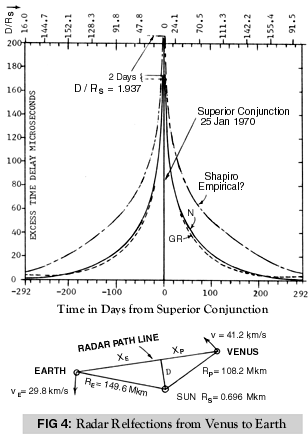Some people say they cannot visualise the concept of negative mass. This is very easy in fact because an object made from it would look exactly the same as one made from positive mass. To illustrate the point, two identical rockets are shown in Fig. 4. The upper one refers to a system in positive mass and the lower to one in the negative kind. In the upper the gas jet reacts to provide a positive accelerating force "F". This balances the rate at which momentum is being carried away by the jet. It leads to a positive acceleration "a" of the rocket. The latter has a mass "m" and after acceleration to a speed "v" the rocket possesses a positive momentum "p" given by mass times velocity or "p = m.v". For the lower case the jet of negative mass produces reversed force of reaction, which is therefore negative and can be written "-F". But this acts on a rocket of negative mass "-m" and so the acceleration produced is given by:- "a = -F/-m" and is identical with "F/m". Hence the acceleration produced has the same direction as before. The momentum of the rocket on reaching speed "v" is, however, now "p = -m.v" and so is negative. Negative energy of motion, negative kinetic energy, has also been gained. Furthermore energy and mass are equivalent as proved by the extension to Newtonian physics given in Chapter T.S.2. It gives a derivation which parallels the one for which Einstein is famous but owes nothing to relativity. For the case of the rocket in negative mass this yields the equation "E = -m.c2" and so the mass-energy, or equivalently, the "rest-energy" of the rocket is negative. It exists in a negative energy state. A negative pressure needs to be applied to accelerate the jet so that it tends to pull the walls of the combustion chamber inward. The atoms of the wall, made in negative mass, however, respond by accelerating outwards. Hence the chamber tends to burst, just as in the case of the system in positive mass. A pressure gauge made in negative mass would therefore give a Positive reading. However the comparison is made, identical responses are found. It is therefore impossible to know whether any system is positive or negative! When Newton formulated his laws of motion he only assumed that the direction of the accelerating force was the same as that of the response, but he did not know this was the case. This is because, at the sub-atomic level, the direction in which the force of action points cannot be determined. It may seem that it can, looked at from a superficial level, but at a deeper level this is readily shown not to be the case. For example, if two billiard balls strike one another they bounce apart. It is natural to jump to the conclusion that the sub-atomic particles, which come into contact, push against each other to create the observed response. But if the objects were made from negative mass and the contact forces pulled away from each other, the same response would arise. Only responses can be observed, hence the direction of the interacting forces and the sign of the masses involved is fundamentally indeterminate. All that can be deduced is that the interacting masses had the same sign. FIG. 14* (T.S.) illustrates the acceleration of objects made from the two kinds of mass and may help in visualisation if the picture is not yet clear. * See page 282. Newton may not have realised that the negative option was available. There is in consequence a fifty per cent chance that he could have been wrong in assuming that our mass system is positive. Our system on Earth could easily correspond with the negative case. If so it does not matter and so there is no justification for not letting things stand as they are. The only time anything strange can occur is when negative mass impinges on the positive. Then, as already deduced, an attractive response will arise. If a billiard ball could be made of negative mass and be projected against one of the positive kind, then instead of the latter being bounced forward, it would bounce back. It cannot be said that this response has not been observed. An object charged with negative electricity is attracted toward a positively charged object. This happens just as well in pure vacuum as in air. The quantum explanation used here is that tiny particles, the mediators of electric force, are being thrown off as virtual negative mass from each and interact with the other. Mediators need to be of the virtual kind, which means they exist on borrowed energy. Then they can carry momentum to transmit forces between elementary particles without causing them to gain or lose any of the energy from which the mediators are made. It needs to be emphasised that negative mass/energy and negative electric charge are two totally different things which must not be confused. But just as both kinds of electricity are needed to structure matter, so both kinds of mass and therefore energy, must also be involved. Atoms need to be composites of both kinds. Then the observed mass of an object will be the net value, the sum of the positive and negative components, according to the extended Newtonian physics. The electrons and nuclei can each be considered to have net positive mass. Then the mediators which bind the electron to the nucleus to make an atom will be of the negative kind and cancel out a large fraction of positive mass. In the TECHNICAL SUPPLEMENT a condensed derivation of the way the Newtonian physics has been extended to provide a successful theory of quantum gravitation is given. The features important to the present argument will be summarised. The first is that the building material of the universe is energy, which exists as the "rest energy" of stationary objects, to which is added "kinetic energy" when in motion. The sum of the two is defined as "total energy" and no potential energy, that due to position in the field, is included. These energy forms exist in both positive and negative states. Carriers of negative momentum have already been shown to be made from negative energy. All these forms of energy can have an alternative representation as an equivalent amount of mass, related by Einstein's well-known equation: E = m.c2 Paul Dirac about 1930 was the first to point out the Possible existence of negative energy states(202). He considered space to be filled to capacity by electrons in negative energy states. Then a high concentration of Positive energy could change the sign of one of them to create a real electron apparently from nothing. This model explained how an energetic photon passing close to a heavy atomic nucleus could turn into an electron positron pair but the explanation is now known to be incorrect. However, a considerable body of literature concerning negative mass has accumulated since that time. Because of the controversy surrounding this subject an entire reference section is allocated to it. These are numbered (301) to (314). Some of the previous descriptions regarding the nature of negative mass are supported by this literature. The exact symmetry of the two kinds leading to the conclusion that it is not possible to tell which is which appears to be new. Also Forward(308) and Will(108) show the gravitational force on negative mass oppositely directed to what we will predict. At present physicists dismiss the possibility that negative mass could exist, mainly on the grounds of incompatibility with Einstein's theory of general relativity! There are some other objections but they are also inapplicable for the extended Newtonian physics. There are indeed a number of reasons why negative states are an embarrassment to standard quantum theory and so followers of the establishment use them to justify their rejection. In consequence, however, they have no valid way of representing attractive forces. As already mentioned they adopt the stratagem of the "negative coupling" instead. This, however, is no more than an artificial reversal of direction of force to make the answers come out right. The negative coupling idea cannot be logically justified because it leads to an internal contradiction. An arbitrary volume of space can be marked out to surround the absorbing particle. This space acquires an increment of positive momentum as any mediator of attractive force enters this volume. Then on absorption, due to negative coupling, the same volume is instantly switched to one containing an element Of negative momentum. This is a logical impossibility! One reason for the total failure of theoreticians to achieve a satisfactory explanation of quantum gravitation has therefore emerged. The reason for our detailed analysis of negative energy states to "start the ball rolling" should now be clear. The main objection mathematician's raise is irrelevant in the present theory and the others are readily countered. For example, Paul Davies(106) describes one of these. The nucleus of an atom is surrounded by electrons whose speed and position determine their energy levels. They tend to fall from higher to lower energy levels by emitting energy differences as the photons of light. Indeed this is the way light is produced. If, therefore, the argument goes, negative states can exist, then electrons would fall through zero, go negative and fall forever. Because this does not happen negative states cannot exist. To counter this all that is required is the postulation of an exclusion principle. Electrons can only emit energy of the same sign as themselves. Electrons of negative energy cannot then emit photons of positive energy. Then they can never fall through the zero energy state. The only other relevant objection, which has been thrown against the new theory, is that if matter is a composite of the two kinds of energy, it would be unstable and would annihilate itself. However, in the new explanation of wave-particle duality, yet to be described in the next two chapters, this is required to happen all the time. It is a part of the new theory and is therefore not an objection at all. It seems that all real elementary particles need to be surrounded by a protective barrier able to at least delay mutual annihilation and at the same time prevent the reverse process, the emission of an opposite energy state. It was my hope that the lecture delivered at The University of Leeds in January would lead to a publication of the present theory in a scientific journal. Their specialist in gravitation objected, however, largely on the grounds that negative energy states were incorporated. I quote from his letter of rejection: "As a matter of fact, negative energy states are a severe embarrassment. Indeed one of the great advantages of quantum field theory (from which the notion of virtual particles arises) is that it enables us to reinterpret Dirac's quantum mechanics (and other related theories) in a way which does not involve negative energies." Yet Professor Stephen Hawking has received much acclaim for his concept of "Hawking Radiation". Inside "Black Holes" the gravitational force is predicted by general relativity to be so huge that nothing can escape, not even light. Yet according to Hawking they radiate positive energy and so gradually evaporate. The radiation depends on space containing particles in pairs. Some fall into the hole leaving their partners to fly off as radiation. Those falling in cancel an equal amount of positive energy at the centre because they are built from negative energy states! So another inconsistency exists in quantum theory! If negative states are excluded in some areas because they do not fit in, then they must be excluded altogether. The question needs to be asked, "Is the embarrassment to other areas of the theory due to some fundamental wrong assumption?" There are other difficulties, such as the problem of a predicted "Cosmological Constant" in established theory, which is fifty orders of magnitude greater than astronomical observations can possibly allow! Again this vanishes when the existence of negative states are permitted, as will shortly be seen. We shall continue on the assumption that it is reasonable to include them in the new theory. It is then possible to turn attention to fundamental questions such as the energy balance across the creative event of the universe. Negative and positive energy forms in equal amounts, crushed together, would mutually annihilate, leaving nothing. Creation of the universe would be the converse case, nothing giving rise to positive and negative energies in equal amounts. The "Yin" and the "Yang" Of energy, needing each other absolutely for their simultaneous creation! In this way the universe could have been created ex-nihilo. In the beginning the raw material of construction had to be pure nothingness. Established theory recognises this necessity but adopts a different interpretation. In this, as argued by Tryon(123), gravitational potential energy (GPE) is assumed to represent negative energy. He shows the amount available to be roughly equal to the energy of all matter in the universe. Hence the two could cancel to zero. The assumption that GPE is negative, however, is open to serious doubt. It is based on measurement from an arbitrary datum, taken at infinite distance. As the galaxies fly out and eventually come near to a stop at infinity, their mass-energy will still exist. it is most unlikely that matter would progressively disappear as it approached this arbitrary zero point. Furthermore, if an object falls from infinity, it speeds up as its GPE reduces. Then if the excess kinetic energy is removed in some way, the object will orbit the mass to which it was attracted and its GPE will be negative as compared with the zero value it had at infinity. This is the justification for saying that GPE is negative energy. But the datum was fixed at infinity only for convenience of calculation and it could with equal validity have been taken at any other place. It could have been taken, with greater justification, at the place where matter originated in the assumed "Big Bang" of creation. In this case however GPE appears as positive energy. As the bits fly out from the centre in the violent explosion which must have happened, they gain in positive GPE as the speeds fall. A careful analysis of this proposal suggests, therefore, that this cannot be a valid proposition. But the idea raises an important question. Is gravitational energy positive or negative or even real energy at all? We will not try to answer this question yet. We will leave it to Chapter 11, "FUTURE PROJECTIONS", where a most exciting answer will emerge! An internal contradiction in the standard "Big Bang" theory of creation also came to light in the introductory chapter. All the energy of the universe arose from nothing in a split second as a massive violation of the First Law of Thermodynamics yet this law has been obeyed exactly ever since. A resolution of the difficulty now seems to be coming in over the horizon. It depends on accepting negative energy states. Then the creation can arise from a zero energy state without needing negative gravitational potential energy. The permanence of energy after the event will soon be seen to be an illusion, which nevertheless is adequate for practical purposes. The concept adopted here is amenable to mathematical treatment and leads to exciting new developments. The totality of everything is made from exactly balanced forms of energy. There is no need to invoke GPE to create a balance. 5.4 Quantum Gravitation - The New Solution In the new theory the matter of our universe has a net positive energy whilst space, empty of matter, consists of balanced positive and negative kinds. These are composed mainly of the mediators of repulsive and attractive forces respectively. The net energy of all matter is balanced by an equal net negative energy superimposed on the other energies of space and spread over a huge volume. For condensed objects, such as stars, the balancing negative energy forms a tenuous halo stretching out for about a billion light-years in all directions. It consists of virtual particles, continually generated at the surfaces of all real particles to stream out in all directions. Ultimately these virtual particles reach the end of their lives and expire, so that equilibrium always exists to maintain a stable halo. If mediators have no energy of their own, it may be asked, then how could they add up to yield a permanent energy for the complete halo? The answer is that they each possess energy for their lifetime. They arise from nothing, have a life, then vanish. Because of the lifetime a halo of permanent energy exists, just as people who have finite individual lives maintain the population of a country for an indefinite time. Each mediator exists on a debt needing to be balanced by positive energy, so a succession of them allows matter to exist. Not only stars have gravitational haloes. Planets will have them and so will smaller objects. All objects will have them, including humans, becoming ever more tenuous as distance increases and stretching for about a billion light years in all directions. As mediators spread out with distance, the density of the halo diminishes according to the inverse square law. This is illustrated in FIG.2* of the T.S. Being of negative energy, the virtual particles of the halo are carriers of negative momentum and so exert a universally attractive force on all positive matter in their path. They provide the primary force of gravitation. Clearly, if mediators are generated and intercepted by matter in proportion to the mass of objects assumed to remain constant, then Newton's inverse square law of force will be predicted by quantum theory. *See Page 254 At this point it is necessary for the reader to accept that negative as well as positive energy states can exist. The further development of the explanation for wave-particle duality is dependent upon this acceptance. The remainder of this chapter is a description of the new theory of quantum gravitation, however, and is not essential as far as an explanation Of Psychic forces and the paranormal are concerned. The new solution gives further support for the existence of negative energy states by showing how the existing experimental checks can be met. Many readers will, I feel, be interested to see how the problem of quantum gravitation can be resolved. Others might wish to jump straight to "5.6 Conclusions Regarding Negative Energy States" at this point. 5.5 Quantum Gravitation - More Detail Coming back to our theme, it is known that Newton's inverse square law of force does not apply exactly to the force of gravitation and his theory gives some other wrong predictions. The differences have resulted in Einstein's theory of general relativity becoming established. One important effect is that Newton's law predicts that a single planet in orbit around a relatively massive star would move in a perfectly elliptical orbit. The observation of Clemence(204) for the planet mercury showed, however, that the axes of the ellipse also rotate slowly. This effect is known as "Precession of the perihelion" and is illustrated by the half -orbit shown in FIG. 12 (T.S.). Einstein's theory predicted the correct result almost exactly and was proclaimed to be a major triumph early in the century. In the extended Newtonian physics, however, a small but highly significant modification is introduced. it is deduced, as a consequence of light falling like matter in a gravitational field, that the rate of mediator emission and interception must be proportional to total energies of the objects interacting gravitationally, instead of their rest masses. Total energy, it will be remembered, is the sum of rest and kinetic energies with potential energy ignored, in the present theory. Since the kinetic energy of objects in a state of free fall increases as the separating distance from a ponderous mass reduces, the total energy of a planet is greatest at the point of closest approach. Hence the inverse square law of force is modified, being steepened slightly. The mathematical evaluation summarised in the T.S. gave an equation which looks quite different from the one Einstein deduced. These are compared in FIG.13(T.S.) But as also shown in this illustration, it gives exactly the same precession for any conceivable orbit within the solar system! Sufficient information is included in the figure for the reader to carry out independent checks. The magnitude of the force could be calculated from the total mass of mediators in the halo, provided the range of gravitation could be specified. It may be argued that the range is known to be infinite. This is not true. It has only been assumed that the range is infinite in theories which have only been partially successful or which are abstract like Einstein's general relativity. He makes no attempt to provide a mechanism able to account for the existence of a force of gravity. Indeed his theory actually says no such force exists. This is one of the main incompatibilities of relativity and quantum theory, because the latter demands that a force exists. At least it did so in its original formulation before people tried to alter its concepts to fit in with Einstein. The extended Newtonian physics goes back to the original concept that forces have to exist in order to cause acceleration. This is demanded now that the concept of space curved in higher dimensions has been abandoned. This argument therefore justifies the idea that gravitation has a large though finite range. A lower limit can be guessed from the size of superclusters of galaxies. Galaxies of stars are structured by gravitation, most appearing as flat spirals in slow rotation. Then galaxies are grouped into clusters and the clusters into superclusters. This sets the minimum range at about a billion light-years. Now since the mediators travel outward at a constant rate, their mass contained within any unit of distance measured radially outward will remain constant. The total will therefore equal their energy density measured at the surface of the Planet or star of origin, multiplied by the surface area of that emitting object and the radial distance mediators travel before expiry. Since their total energy must equal that of the object they balance, it is easy to work out the density of the halo at any point provided the range is known. If it is further assumed that gravitational mediators travel at the speed of light, then the condition giving the maximum possible gravitational force will have been assumed. Using the method previously outlined, the predicted gravitational fore turned out to be several orders of magnitude too low Hence some form of amplification of the primary fore needed to be in operation. The amplifying factor turned out to be space itself! The huge but exactly balanced energies of the bulk of the particles of space also interact with the primary mediators. The latter cannot therefore be imagined a travelling in straight lines. Instead they diffuse through space, bouncing away from other particles of space in their path, so zig-zagging about. Mediators will drift in the direction of decreasing concentration just as molecules of one gas diffuse through another. The interactions cause space to be compressed in the vicinity of ponderous masses, rather in the manner of the gaseous atmosphere held by gravity around a planet, though with density tailing off more gradually with distance by many orders of magnitude. It is possible to imagine uniform or undistorted space by thinking of it as composed of virtual particles arranged on a cubic lattice. One particle appears at the centre of each of a large number of imaginary cubes of equal size stacked together. They all have a common separating distance "L1" equal to the lengths of the edges of the cubes. In compressed space all values of "L1" are equally reduced to a smaller one "L". In fact the virtual particles are always spontaneously appearing and disappearing at random. Hence both "L1" and "L" are really average separating distances; no cubic lattice distribution could be observed in practice. It is assumed that the size Of each cube is so taken that within it a new particle will arise somewhere just as its predecessor expires. Then energy inside each cube can be regarded as remaining in an uninterrupted and constant state at all times. Each cube contains a sequence of virtual particles joined end to end in time. This might be termed a "particle sequence". The model is illustrated in FIG.8 (T.S.). This now represents the model of space described by Novikov(211), though he only admits of the existence of positive energy.  The energy of the particle sequence in each cube will not be affected if the volume of the cube is reduced by compression. The situation is therefore slightly different from that of a gas made up of permanently existing molecules. In this case the energy will rise. The term "energy density" is, however, defined the same way. It is the energy content divided by the volume of its containing cube. Hence for space, even though the energy of the particle sequence remains fixed, the energy density increases when the cube is made smaller. So the energy density of space increases as space is compressed. Half the virtual particles of space will be of positive energy, with the other negative. Each half can be thought of as separate entities interpenetrating one another and each having its own energy density. And associated with each energy density is a pressure just as in the case of an ordinary gas. When space is more compressed in one place than in another, then an "energy density gradient" exists between the two, just as a slope is needed to connect the bottom of a hill to the top. A pressure gradient is induced in space proportional to the consequent energy density gradient. Then this Pressure gradient acting across the volume of each elementary particle produces an effect like a buoyancy force. The positive half of space will push elementary Particles upwards, just as a cork is pushed upwards in water, but this effect needs to be more than cancelled by the opposite effect of the negative half pushing downwards. Elementary particles need to be considered as soft rather than hard so that the virtual particles of space penetrate to varying degrees when they interact. Then the volume "seen" by the negative half of space can exceed that of the positive half. In this way the net attractive force produced by the primary Mediators can be amplified. The same imbalance of apparent particle size is also needed to secure the compression of space. As mediators (of negative energy) diffuse through space, negative particles will bounce away whilst positives move toward impinging mediators. For a net compressive effect to arise it follows that the apparent size of the positive. must exceed that of the negatives. Hence a general law can be established which states that: "When elementary particles impinge upon one another, whether virtual or real, the effective volumes are greater when opposite energies interact than when similar energies interact." This leads to the prediction that if sub-atomic particles are positive/negative composites, then the gravitational forces will act in opposed senses on components of opposite mass. Then the net force will be proportional to the net mass, which agrees with observation. But both kinds of mass, when isolated, accelerate in the same direction in a state of free fall. Both kinds of matter will create exactly the same space compression and so all matter will be attracted toward all other matter regardless of whether it is positive or negative. This prediction is at variance with that given by both Will(124), (108) and Forward(308), who say that positive matter will be repelled by negative. This difference is due, however, to the new idea of gravitation arising mainly as a type of buoyancy force. As will be shown, this concept stands a very good chance of being the one which is correct. In the limiting case the volume presented by a real object to the positive half of space is assumed zero. Then it works out that the gross energy density of space, the value obtained when the halves are added with the negative sign ignored, is 1041 J/m3 to give the correct value of gravitational force. This compares with the value of 1045 J/m3 given by Starobinskii and Zel'dovich(215), determined in other ways. The latter value is also several orders of magnitude greater than the energy density of electrons. Hence sub-atomic particles need to be considered as fluffed out forms of energy similar to air bubbles in water. The energy density of mediating particles, however, must obviously be greater than twice the average for the positive half of space, otherwise they would have no room to move. If a factor of 100 is guessed, then it follows that to match the average energy density of space the apparent negative volumes presented by particles need to exceed the positive ones by one part in about 100. This seems a very reasonable result. In Chapter T.S.3 a model for the electric force is derived which requires almost the same energy density for space as the value given by Starobinskii and Zel'dovich. Hence it can be claimed that, for the first time, the ratio between the gravitational and electric forces has been predicted by a theoretical model. This matter will be considered in more detail a little later. The volumes of elementary particles need to be proportional to their total energy in order to give the correct value for the precession of planetary orbits. Hence the energy density of these particles has to be a new universal constant of nature. Again this is a very satisfying conclusion. In this way also the law of force remains the same as that produced by the primary mediators of gravitation first considered. In consequence space compressibility does not affect the way planets exhibit precession or alter the predicted values. A model of space on this basis is illustrated in FIG.15 (T.S.). It shows the halo surrounding a neutron star and the resulting compression of both halves of space. The circles shown represent an elementary Particle subject to buoyancy forces. The upper circle, representing the volume presented to positive mediators, is smaller than the lower circle for the negative ones so that the net effect is a force of attraction. Both Circles, it is to be understood, represent the same Particle. This model of space led immediately to an unsought bonus. One to which allusion was made a little earlier. The electric force, according to quantum theory, also depends on mediators. In the new theory it requires a high energy density for each half of space. Then a coupling scheme for mediators can be worked out to explain how like charges repel whilst unlike charges attract one another. This will be discussed in more detail in Chapter 9 and is illustrated in FIG.6. More importantly for present considerations is the match of energy densities for space previously mentioned, obtained separately to satisfy the electric and gravitational forces. It follows that the magnitudes of these two forces have been related to one another to a fair degree of accuracy. Einstein spent many years attempting to relate the magnitudes of these forces and finally had to give up. Hence in this respect the new theory achieves what general relativity falls down upon. But there is more to come. A horizontal beam of light is bent by gravity as shown in FIG.1* (T.S.). Because light has to travel faster on the outside of the bend, this means that the speed of light varies with level. The compressibility of space adds a doubling effect to this variation. This is because the average spacing of virtual particles has been reduced at a lower level and the distance light travels in a given time is proportional to this average spacing. The photons jump from one virtual charged particle of space to another with a dwell at each in the new theory. As described in Chapter T.S.1 this permits a quantum explanation for the electromagnetic wave to be advanced as a composite structure in which uncharged photons interact with the charged virtual particles of space. This wave is treated in an abstract way in textbooks without attempting to show how the photon can be fitted into the picture. * See Page 253 This structure results in the gravitational deflection being doubled as compared with the value predicted with space compression ignored. The end result is to give an exact parallel with Einstein 15 theory of general relativity. Both theories therefore match astronomical observation equally well. Both give double the deflection of light as compared with the Newtonian prediction. The new theory therefore exactly parallels the achievement of general relativity in this respect. Light travels more slowly at lower levels in a gravitational field according to the extended Newtonian physics. So it takes slightly longer for a beam to travel from one planet to another if the light passes close to the Sun than for the same distance free from the sun's influence. This is the "Shapiro time delay". Again the predictions agree well with observations made during the space exploits to Mars using data reproduced by will (124). A comparison with other theories is given in FIG.10* (T.S.) * See page 273 For the same reason a gravitational red shift is also predicted. This means that a very compact star like a white dwarf, having the mass of our Sun compacted into a sphere no bigger than the Earth, would look redder than it should. The frequency of all vibration has been reduced due to the low level in the field. Yet even this is a weak field from the mathematical point of view. The resulting equation for weak fields is exactly the same as that given by general relativity. Again the new theory therefore matches the experimental checks which have been made. It may be easier to understand this effect by looking at it a different way. The speed of light reduces as level falls yet, as is shown in Chapter T.S.2, the equation "E = m.c2" can be derived from Newton's theory of acceleration without reference to relativity. If "c" falls then "m" must increase to compensate if energy remains constant. Hence it needs greater inertial mass to represent a given energy at a lower level. The increased inertia also means a slower vibration for a given spring, and the red shift worked out this way gives exactly the same result as before. The two methods are exactly consistent with one another. There is another important implication. Particles need to be considered made from energy rather than mass, because in raising or lowering an object on a cable, the rest energy of the object will remain fixed but the corresponding mass will vary. This is why energy in the extended theory, replaces mass as one of the dimensions of the original Newtonian theory. The new theory also predicts that gravitational waves will be generated by rotating dumb-bells. At a great distance a force is predicted to arise, pushing any mass in a direction at right angles to the direction of wave propagation, but the frequency of oscillation produced will be twice the rotational speed of the orbiting dumb-bells. Unfortunately the energy loss by a close pair of stars forming such a dumbbell has not yet been formulated. I have to admit myself stuck on this problem at present. I am still looking for a "handle" to see how to make a start. Hence in all respects except the last-named, the achievements which enabled general relativity to become so firmly established are paralleled and the new has the edge by offering an explanation for the huge difference in the magnitude of the gravitational and electric forces. At the same time the new theory is free from inconsistencies and false prediction whilst the established approach fails on both counts. One big problem arising when quantum theory is joined to general relativity is the prediction of a huge "cosmological constant" which is associated with a force increasing with distance. The predicted force is so huge that, if it existed, the galaxies would be blasted apart at a rate fifty orders of magnitude greater than astronomical observations could possibly allow! Gibbons, Hawking and Siklos (207) state in their workshop proceedings of 1982 that this persistent difficulty undermines confidence in established theory. This false prediction arose from attempts to match quantum theory, which denied the existence of negative energy states, to general relativity so that the combination described a real force. The quantum vacuum, as already described, needs to possess immense energy density. But at the same time it needs to be zero otherwise space could not expand. The solution has been to endow space with a huge intrinsic "negative pressure" of the vacuum. Pressure has the same units as energy density and so from a superficial viewpoint there seems no reason why the two should not be equated to make the net energy of space zero. There is also a pressure term in Einstein's equation for the density of space. When the negative pressure of the vacuum is inserted this has a dominant effect and yields the huge cosmological constant which is still such a source of concern. Physicists have accepted it to be real and are currently looking to other effects to cancel it out. The favourite at the moment is to assume other universes exist in higher dimensions. They have equal but opposite cosmological constants which fortuitously cancel by communication through "wormholes" in space. The theory is described by Abbot(101) although it seems to have been first proposed by Sidney Coleman. In the new theory the problem never arose. The weak universal attraction of primary mediators appeared instead to provide a cause for gravitation. The reader can judge which approach seems the more reasonable. Another problem arising in established theory is associated with the "Black hole" predicted by general relativity. The speed of light falls to zero at a finite radius. But it is still slowing inside although it has already stopped which is logically impossible. Hence the laws of physics break down at this point. Also the universe arose inside a Black Hole. Not even light could escape so the universe could never have emerged! Furthermore, the dynamics of the Big Bang have been worked out using physics in a region where logic has broken down. Some people will counter this by saying that the universe extends so far that we are still inside that Black Hole; there is no need for matter to have emerged. In this case, however, we exist in a region where the laws of physics do not apply! However one twists and turns the Black Hole appears as an embarrassment. Yet students of physics are expected to take it all on board as if it were truly comprehensible. Is it not more reasonable to consider it a pointer to a possible false initial assumption? In the extended Newtonian physics the speed of light only falls to zero at zero radius, no matter how great the concentration of matter assumed at that point. No breakdown of logic arises anywhere and both matter and light can escape in the Big Bang. The Black Hole does not really exist. There is no longer a problem! There are also two very good reasons why the long-range force of gravitation should depend primarily on buoyancy type forces rather than on the absorption of mediators. Firstly, the deeper layers of a planet would be partially shielded if some of the mediators were absorbed before reaching the surface. Hence the absorption models are inconsistent with the gravitational force being proportional to the amounts of matter involved. Also, during eclipses of the Sun by the moon, a gravitational "moon shadow" would arise. These have been looked for without success. No shadow exists. In the buoyancy models mediators are not absorbed, so they are not used up. No shielding effects can therefore arise. The other reason has to do with the conservation of the orbital energy of a planet. This limitation affects the case for the electric force in just the same way as the gravitational case. Mediators streaming radially outward from the Sun will appear, as observed from the planet, to arrive in a slanting direction owing to the "relative wind" resulting from orbital speed. if they are absorbed, then it follows that a tangential component of force will be present. Since an attractive force is being produced by virtual particles of negative energy, this will not produce a drag force. Instead the planet will be pulled forward. It will gain angular momentum, the product "m.v.r" and spiral out of orbit. This does not happen and the force is several orders of magnitude higher than could be balanced by the drag of interstellar gas. Hence there is a serious flaw in the model. With the buoyancy type of force, however, no tangential component of force arises and so no such problem exists. Some problems of relating the buoyancy type of long-range force to the short-range forces now appear however and these need resolution. The latter are the strong force which structure the atomic nucleus and the weak force responsible for radioactive decay. The long range force of gravitation can be considered first. If a star is non-rotating, then the mass of the star will exactly equal the sum of the parts assembled from infinite distance. This is because mediators are not being absorbed. Also if an object is lowered on a cable, then any work done by motion in the field due to the induced weight is balanced by an opposite force on the cable. The energy released by lowering is transferred to the fixed lowering device which pays out the cable. Hence no net energy transfer is able to arise to change the rest-energy of the object. So the rest-energy remains constant when an object is moved to any point in the gravitational field. The electric force and that of magnetism have already been related by abstract reasoning and are considered as the single force of "electromagnetism". This long-range force also needs to have a buoyancy force basis if tangential force components are to be absent. Here in the new theory the force is caused by the partial energy density gradient acting across the volume of a particle. Such gradients are many orders of magnitude greater than those of space as a whole and give about the correct ratio of gravitational to electric force. This matter is dealt with in detail in Chapter T.S.3 which includes FIG. 24* illustrating what is meant by partial energy densities. * See Page 356 On this model an electron falling from a great distance in the electric field of a naked atomic nucleus will speed up. If it is aimed to miss the nucleus, then it will reach a point of closest approach and fly out again. This can only be prevented by causing the excess kinetic energy gained during the fall to be radiated away. The rest mass and energy will remain constant at all points within the electric field because the same argument as that used for gravitation will apply equally. But some kinetic energy remains in order to maintain a stable orbit. It follows that the mass 0 the atom will be slightly larger than the sum of the separated parts measured at rest. A similar result applies to a rotating star. Both these long-range forces have this property in common, which means that neither the electric or gravitational binding energies are reflected by mass changes. This is contrary to the established view. However, the mass differences involved are so small that it would be quite impractical to resolve the issue by weighing. Hence no experimental evidence exists which could discriminate between the two different predictions. Supporters of the establishment case are likely to claim that for the strong nuclear force mass difference have already been measured and are known to accurately reflect binding energies. The mass of any nucleus is always slightly less than the sum of the parts measured before assembly. Hence the electromagnetic and gravitational binding energies will likewise be reflected by mass differences, they will say. The case is likely to be supported by reference to the weak force. The latter is responsible for radioactive decay. Something needs to hit an unstable nucleus in order to make it split. The something has to be a virtual particle arising temporarily from the quantum vacuum to be absorbed by that nucleus. Hence the case for mediator absorption rather than the buoyancy force model is strongly supported on two counts. This argument is likely to be used to discredit the new buoyancy model unless a good case can be made out showing this deduction to be false. And it can! It simply means that the strong and weak forces, which are of very short range, depend mediator absorption whilst the long-range forces do not. Two nuclei coming within the short range of the strong attractive force will exchange mediators which are virtual particles of negative mass. By absorption negative momentum will be exchanged, causing the nuclei to accelerate toward one another, so gaining kinetic energy. But the mediators have no permanent energy, so momentum is exchanged without causing any change of total energy. It follows that in this case some of the rest energy must convert to the kinetic form. Hence the rest energy of the nuclei must fall as they approach. Then after radiating away excess kinetic energy, the sum of the masses will be less than that of the separated components. Now the binding energy of the nucleus will be reflected in mass differences. But the absorption model, when applied to both long-range forces, showed that the objects would experience tangential forces, causing them to gain angular momentum continually. This indeed was one of the two reasons the absorption model had to be rejected. However, a new factor emerges in the case of the strong force which prevents this effect. The objects will now orbit one another at speeds close to that of light and they are of similar masses. Conditions can then be found at which stable states apply with neither component gaining any tangential speed. The same argument can be extended to show why the electron must have the fixed spin which quantum theory demands and this is explained in Chapter T.S.3. Such conditions cannot apply in the case of very dissimilar masses orbiting one another at speeds low as compared with light. Hence it seems the long-range and short-range forces can have a different basis, the former of buoyancy kind and the latter of absorption. Yet all can still be described by a common quantum theory because in the buoyancy case mediators are still involved. In this case, however, they bounce away from particles instead of being absorbed. It may be objected that quantum physicists have already successfully related the weak force to the electromagnetic force by a common absorption model. It turns out, however, that if the absorption model for the electric force is substituted, almost the same energy densities of space are needed to provide the mediators. The result can therefore be interpreted to mean that a parallel mathematical solution exists. The correct one must be chosen from a study of any incompatibility 0, false prediction arising. The new approach seems to have the advantage in this respect and so may be pointing the way to achieving a satisfactory "Grand Unified Quantum Theory". 5.6 Conclusions Regarding Gravitation and Negative States Einstein's theory is held by all experts in the field to be the best theory of gravitation in existence. This is the conclusion reached by Davies(108), Will(124) and almost every other theoretician researching cosmology. Yet as shown in the quotation given at the end of Chapter 1, Einstein himself doubted the validity of relativity. But the experimental data supports the new theory equally well, yet at the same time all the difficulties are avoided. And as a bonus the new theory relates the magnitude of the electric force to that of gravitation to within striking distance. This is beyond the scope of relativity. Hence the new rival needs to be taken very seriously indeed! A new theory of gravitation has been described which depends upon space acting in the manner of a compressible fluid operating free from frictional effects at the sub-atomic scale. Fluid friction is measured in terms of "viscosity" and an ideal frictionless fluid, synonymous with one of zero viscosity, is known as a "superfluid". Only one such fluid is known and this is liquid helium. Once set in motion it carries on moving indefinitely. The electromagnetic wave needed to propagate relative to local space in order to be consistent with the new theory of gravitation. This was also a necessary condition for the introduction of a physical model able to account for such a wave. It is, however, incompatible with special relativity. A modified theory of special relativity had therefore to be formulated which was capable of satisfying the same experimental checks without involving any kind of contradiction. Again this is achieved, as shown in Chapter T.S.2, by extending the concept of space as a superfluid. This time it is superfluid on both the small and the large, scale, even including the galactic range of size. it appears from a study of the literature that the idea of treating space as a compressible superfluid has not previously been considered. It is another fundamental difference in the theoretical approach. It is most unfortunate that so many points of disagreement with established theory have turned up. My hope is that these differences will not continue to prevent the new ideas outlined from being communicated. They ought to be discussed and criticised in a constructive way, particularly by theoreticians in the field of gravitation. It could be that a false trail is being followed by the establishment and people have a right to see alternative proposals so that they can judge the issue for themselves. Only in this way can science make progress. The new theory is dependent on the existence of both positive and negative energy states acting in harmony Without the pair only a partial explanation can be provided. Since the complete theory is so successful, the conclusion that negative states exist is strongly supported. This important deduction will now enable us to develop the idea of the Grid further in our search for a complete theory, inclusive of a meaning for psychic energy. Chapters Contents / Notes / Synopsis / Acknowledgements / Background / Chapter 1 / Chapter 2 / Chapter 3 / Chapter 4 / Chapter 5 / Chapter 6 / Chapter 7 / Chapter 8 / Chapter 9 / Chapter 10 / Chapter 11 / Chapter 12 / Chapter 13 Home / Intro / News / Challenge / Investigators / Articles / Experiments / Photographs / Theory / Library / Info / Books / Contact / Campaigns / Glossary

© The International Survivalist Society 2001

Website Design and Construction by Tom Jones, Graphic Designer with HND